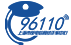2023年01月05日 07:47

编者按：2022年12月12日，深证100ETF期权(标的为深证100ETF，代码159901)上市交易，目前深市共有4只ETF期权产品。为帮助投资者系统了解期权产品特征、理性参与期权交易、有效提升风险管理能力，深交所联合市场机构推出“期权入市手册”系列连载文章。今天是第十五期，让我们学习期权的常用交易策略——垂直价差策略吧！

1.垂直价差策略运用场景

(1)判断标的小幅上涨/小幅下跌，利用认购牛市价差/认沽熊市价差策略降低权利仓单腿策略的成本。

(2)判断标的不涨/不跌，利用认购熊市价差/认沽牛市价差策略锁定义务仓单腿策略的最大亏损。

2.垂直价差策略构建

(1)认购牛市价差：买入认购(低行权价)+卖出认购(高行权价)

(2)认沽牛市价差：买入认沽(低行权价)+卖出认沽(高行权价)

(3)认购熊市价差：买入认购(高行权价)+卖出认购(低行权价)

(4)认沽熊市价差：买入认沽(高行权价)+卖出认沽(低行权价)

3.举例说明认购牛市价差策略损益情况

假设期初标的价格S0=4元，行权价K1=3.9元的近月认购期权价格C10=0.12元，行权价K2=4.1元的近月认购期权价格C20=0.02元。通过买入行权价为K1=3.9元的认购期权、卖出行权价为K2=4.1元的认购期权可构建认购牛市价差策略。

情况一：若到期时，标的价格高于行权价K2=4.1元时，假设到期标的价格S1=4.2元，卖出的认购期权具有行权价值，此时卖出的认购期权价值C21=S1-K2=4.2-4.1=0.1元，卖出的认购期权损益为C20-C21=0.02-0.1=-0.08元；买入的认购期权将也有行权价值，此时买入的认购期权价值C11=S1-K1=4.2-3.9=0.3元，买入的认购期权损益为C11-C10=0.3-0.12=0.18元。此时，认购牛市价差策略的总损益为C11-C10+C20-C21=0.18-0.08=0.1元。随着标的的上涨，卖出的认购期权具有行权价值，将亏损S1-K2-C20；买入的认购期权也具有行权价值，将获利S1-K1-C10.此时，认购牛市价差的盈利为：S1-K1-C10-(S1-K2-C20)=K2-K1+C20-C10。

情况二：若到期时，标的价格高于行权K1=3.9且低于行权价K2=4.1元时，假设到期标的价格S1=4元，卖出的认购期权将没有行权价值，C21=0元，卖出的认购期权损益为C20-C21=0.02-0=0.02元；买入的认购期权具有行权价值，此时买入的认购期权价值C11=S1-K1=4-3.9=0.1元，买入的认购期权损益为C11-C10=0.1-0.12=-0.02元。此时，认购牛市价差策略的总损益为C11-C10+C20-C21=0.1-0.12+0.02-0=0元。因此，若到期标的价格在K1=3.9与K2=4.1之间时，买入的认购期权能赚取S1-K1-C10的收益；卖出的认购期权将没有行权价值，价格归零，可获得卖出认购合约的全部权利金C20.此时，认购牛市价差的盈利为：S1-K1-C10+C20。

情况三：若到期时，标的价格低于行权价K1=3.9元时，卖出的认购期权没有行权价值，此时卖出的认购期权价值C21=0元，卖出的认购期权损益为C20-C21=0.02-0=0.02元；买入的认购期权将也没有行权价值，此时买入的认购期权价值C11=0元，买入的认购期权损益为C11-C10=0-0.12=-0.12元。此时，认购牛市价差策略的总损益为C11-C10+C20-C21=-0.12+0.02=-0.1元。随着标的的下跌，卖出认购期权将没有行权价值，价格归零，可获得权利金C20；买入的认购期权也没有行权价值，价格归零，将亏损权利金C10.此时，认购牛市价差的亏损为：C20-C10。

认购牛市价差策略总结：认购牛市价差策略实际上是在买入低行权价认购期权的基础上，卖出了一个较高行权价的认购期权，放弃高行权价之上的潜在收益以换取增厚收益(即卖出期权的权利金)，使得认购牛市价差策略的收益结构为收益有限、风险有限(认沽熊市价差同理，不再赘述)。

4.认购牛市价差策略注意事项

(1)合约数量关系：买入一张认购期权对应卖出一张认购期权。

(2)行权价的选择：买入的认购合约一般选择平值附近的合约；卖出认购合约的行权价可选择投资者判断标的短期上涨的最高价格，或者为标的上涨时，满足投资者短期收益预期的价格。

(3)合约期限选择：考虑期权合约的流动性情况，一般选择当月或者下月合约进行交易

5.举例说明认沽牛市价差策略损益情况

假设期初标的价格S0=4元，行权价K1=3.9元的近月认沽期权价格P10=0.02元，行权价K2=4.1元的近月认沽期权价格P20=0.12元。通过买入行权价为K1的认沽期权，卖出行权价为K2的认沽期权可构建认沽牛市价差策略。

情况一：若到期时，标的价格高于行权价K2=4.1元时，卖出的认沽期权没有行权价值，此时卖出的认沽期权价值P21=0元，卖出的认沽期权损益为P20-P21=0.12-0=0.12元；买入的认沽期权将也没有行权价值，此时买入的认沽期权价值P11=0元，买入的认沽期权损益为P11-P10=0-0.02=-0.02元。此时，认沽牛市价差策略的总损益为P11-P10+P20-P21=-0.02+0.12=0.1元。随着标的的上涨，卖出认沽期权将没有行权价值，价格归零，可获得权利金P20；买入的认沽也将没有行权价值，价格归零，将亏损权利金P10.此时，认沽牛市价差的盈利为：P20-P10。

情况二：若到期时，标的价格高于行权K1=3.9元且低于行权价K2=4.1元时，假设到期标的价格S1=4元，卖出的认沽期权将具有行权价值，P21=0.1元，卖出的认沽期权损益为P20-P21=0.12-0.1=0.02元；买入的认沽期权没有行权价值，此时买入的认沽期权价值P11=0元，买入的认沽期权损益P11-P10=0-0.02=-0.02元。此时，认沽牛市价差策略的总损益为P11-P10+P20-P21=0-0.02+0.12-0.1=0元。因此，若到期标的价格在K1=3.9元与K2=4.1元之间时，卖出的认沽期权具有行权价值，可获得P20-(K2-S1)的盈利；买入的认沽期权没有行权价值，价格归零，将亏损全部权利金P10.此时认沽牛市价差策略的损益为：P20-(K2-S1)-P10。

情况三：若到期时，标的价格低于行权价K1=3.9元时，假设到期标的价格S1=3.8元，卖出的认沽期权具有行权价值，此时卖出的认沽期权价值P21=K2-S1=4.1-3.8=0.3元，卖出的认沽期权损益为P20-P21=0.12-0.3=-0.18元；买入的认沽期权将具有行权价值，此时买入的认沽期权价值P11=K1-S1=3.9-3.8=0.1元，买入的认沽期权损益为P11-P10=0.1-0.02=0.08元。此时，认沽牛市价差策略的总损益为P11-P10+P20-P21=0.08-0.18=-0.1元。随着标的的下跌，卖出的认沽期权具有行权价值，将亏损为P20+S1-K2的价值；买入的认沽期权也具有行权价值，将获得K1-S1-P10的收益。此时，认沽牛市价差策略的损益为：(P20+S1-K2)+(K1-S1-P10)=K1-K2+P20-P10。

认沽牛市价差策略总结：认沽牛市价差策略实际上是在卖出高行权价认沽期权的基础上，买入了一个较低行权价的认沽期权为卖出的高行权认沽期权锁定下行风险，使得认沽牛市价差策略的收益结构为收益有限，风险有限。(认购熊市价差同理，不再赘述。)

6.认沽牛市价差策略注意事项

(1)合约数量关系：买入一张认沽期权对应卖出一张认沽期权。

(2)行权价的选择：卖出的认沽合约一般选择平值或者实值合约；买入的认沽期权合约一般选择虚值合约，选择买入期权行权价时，需要权衡权利金成本和保险效果。

(3)合约期限选择：考虑期权合约的流动性情况，一般选择当月或者下月合约进行交易。

（文章来源：证券时报）